### 5.11  Module Int32

32-bit integers.

This module provides operations on the type `int32` of signed 32-bit integers. Unlike the built-in `int` type, the type `int32` is guaranteed to be exactly 32-bit wide on all platforms. All arithmetic operations over `int32` are taken modulo 232.

Performance notice: values of type `int32` occupy more memory space than values of type `int`, and arithmetic operations on `int32` are generally slower than those on `int`. Use `int32` only when the application requires exact 32-bit arithmetic.

val zero : 'a int32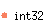The 32-bit integer 0.

val one : 'a int32The 32-bit integer 1.

val minus_one : 'a int32The 32-bit integer -1.

val neg : 'a int32 -> 'a int32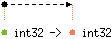Unary negation.

val add : 'a int32 -> 'a int32 -> 'a int32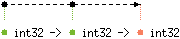val sub : 'a int32 -> 'a int32 -> 'a int32Subtraction.

val mul : 'a int32 -> 'a int32 -> 'a int32Multiplication.

val div : 'a int32 -> 'b int32 -{'c | Division_by_zero: 'c |}-> 'a int32
with 'b < 'a, 'cInteger division. Raise `Division_by_zero` if the second argument is zero. This division rounds the real quotient of its arguments towards zero, as specified for `Pervasives.(/)`.

val rem : 'a int32 -> 'b int32 -{'c | Division_by_zero: 'c |}-> 'a int32
with 'b < 'a, 'cInteger remainder.

val succ : 'a int32 -> 'a int32Successor. `Int32.succ x` is `Int32.add x Int32.one`.

val pred : 'a int32 -> 'a int32Predecessor. `Int32.pred x` is `Int32.sub x Int32.one`.

val abs : 'a int32 -> 'a int32Return the absolute value of its argument.

val max_int : 'a int32The greatest representable 32-bit integer, 231 - 1.

val min_int : 'a int32The smallest representable 32-bit integer, -231.

val logand : 'a int32 -> 'a int32 -> 'a int32Bitwise logical and.

val logor : 'a int32 -> 'a int32 -> 'a int32Bitwise logical or.

val logxor : 'a int32 -> 'a int32 -> 'a int32Bitwise logical exclusive or.

val lognot : 'a int32 -> 'a int32Bitwise logical negation

val shift_left : 'a int32 -> 'a int -> 'a int32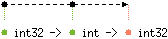`Int32.shift_left x y` shifts `x` to the left by `y` bits. The result is unspecified if `y < 0` or `y >= 32`.

val shift_right : 'a int32 -> 'a int -> 'a int32`Int32.shift_right x y` shifts `x` to the right by `y` bits. This is an arithmetic shift: the sign bit of `x` is replicated and inserted in the vacated bits. The result is unspecified if `y < 0` or `y >= 32`.

val shift_right_logical : 'a int32 -> 'a int -> 'a int32`Int32.shift_right_logical x y` shifts `x` to the right by `y` bits. This is a logical shift: zeroes are inserted in the vacated bits regardless of the sign of `x`. The result is unspecified if `y < 0` or `y >= 32`.

val of_int : 'a int -> 'a int32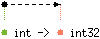Convert the given integer (type `int`) to a 32-bit integer (type `int32`).

val to_int : 'a int32 -> 'a int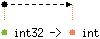Convert the given 32-bit integer (type `int32`) to an integer (type `int`). On 32-bit platforms, the 32-bit integer is taken modulo 231, i.e. the high-order bit is lost during the conversion. On 64-bit platforms, the conversion is exact.

val of_float : 'a float -> 'a int32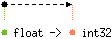Convert the given floating-point number to a 32-bit integer, discarding the fractional part (truncate towards 0). The result of the conversion is undefined if, after truncation, the number is outside the range [`Int32.min_int`, `Int32.max_int`].

val to_float : 'a int32 -> 'a float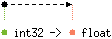Convert the given 32-bit integer to a floating-point number.

val of_string : 'a string -{'b | Failure: 'b |}-> 'a int64
with 'a < 'b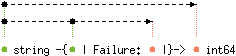Convert the given string to a 32-bit integer. The string is read in decimal (by default) or in hexadecimal, octal or binary if the string begins with `0x`, `0o` or `0b` respectively. Raise `Failure "int_of_string"` if the given string is not a valid representation of an integer.

val to_string : 'a int32 -> 'a string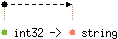Return the string representation of its argument, in signed decimal.

type (#'a:level) t = 'a int32
An alias for the type of 32-bit integers.

val compare : 'a t -> 'a t -> 'a int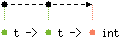The comparison function for 32-bit integers, with the same specification as `Pervasives.compare`. Along with the type `t`, this function `compare` allows the module `Int32` to be passed as argument to the functors `Set.Make` and `Map.Make`.